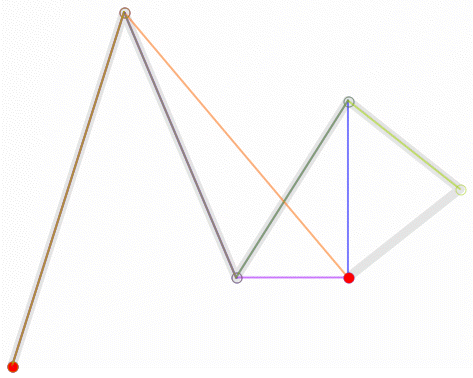A bit of math behind Ant-ManI write also for Lo Spazio Bianco (The White Space), a web-zine about comics. Thanks to Carlo Coratelli, that contacted Sarofsky Corp., we can realize an interview with Erin Sarofsky, President, Owner and Executive Creative Director of the company. They realized some titles for Marvel Studios movies, in oparticular Ant-Man, and I proposed two questions about this movie. The questions, with Erin's answers, are the following, but you can read the complete interview here:
We loved the opening animation in Ant-Man. It seems it's inspired by the Power[s] of Ten of the Eames. Is it really like that?
Ab-So-Lutely! We were very inspired by the Eames film. Luckily though, our universe is the Marvel Universe... so being 100% accurate was not necessary.
About Ant-Man, we also loved the work with the mathematical modeling you used to produce the animation and we think it would be quite interesting to elaborate about the actual realization method. How does it work?
We actually worked in reverse. We planned our moves and then did the math after the fact. We used the surface of the grass as our zero; anything above that is positive and anything below is negative.
Andy Zazzera, CG Director on the job, and our wicked smart math guy did all the estimations. (Again, nothing is exact because our world is fake... but the math is legit).
For a while I was tempted to quest something of more detailed about the math developing, but I thought that it could not so interesting for our regular readers. However, I'm convinced that it is not for Field of Science's readers!
The mathematical basis of 3d modeling originate in automotive industry, in particular thanks to Pierre Bézier that developed the Bézier's curves for Renault, starting from an algorithm developed by Paul de Casteljau for Citroen.
Bézier's curves are defined from two or more control points, $P_i$, and using a parameter $t \in [0,1]$: $B (t) = \sum_{i=0}^n b_{in} (t) P_i$ where $b_{in}$ are the Bernstein's polynomials $b_{in} (t) = \binom{n}{i} t^i (1-t)^{n-i}$ with $i = 0, \cdots, n$.
In the same way it is possible to define the Bézier' surfaces, that are an important tools in computer graphics.Another useful tools for 3d modeling is the continuation method (or numerical continuation). Given a system of equations $F(x) = 0$, you can choose an appropriate system $G(x)$ that is more simple and also a continuous evolution of the system $F(x)$. In other words there must be exist a continuous transformation between $F(x)$ and $G(x)$. This method was refined by Alexander Morgan in 1980s for General Motors:1 comment:

1.Markup Key:
- <b>bold</b> = bold
- <i>italic</i> = italic
- <a href="http://www.fieldofscience.com/">FoS</a> = FoS